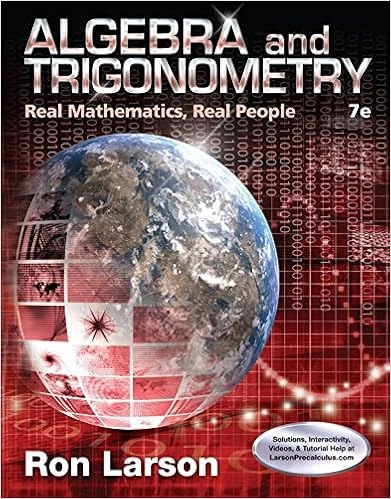# 1 mb 1 megabyte 1024 kb 1 kb 1 kilobyte 1024 bytes 1

• Notes
• 91

This preview shows page 83 - 85 out of 91 pages.

##### We have textbook solutions for you!
The document you are viewing contains questions related to this textbook.The document you are viewing contains questions related to this textbook.
Chapter 8 / Exercise 26
Algebra and Trigonometry: Real Mathematics, Real People
LarsonExpert Verified
1 MB = 1 megabyte = 1024 KB 1 KB = 1 kilobyte = 1024 bytes 1 byte = 8 bits 1 bit holds one binary piece of information (0 or 1) 1 double precision variable requires 64 bits (b) Repeat this calculation for the Cray-2 supercomputer, which has 512 megawords (MW) of memory. Assume that 500 MW is available for our use. 1 MW = 1 megaword = 1024 × 1024 words 1 word = 64 bits (Notice that, on the Cray, the default variable size is double precision.) (c) What is the largest system of equations Ax = b that we could reasonably solve on a Mac SE/30 if computer speed is our only limitation? Assume that we will be using Gauss elimination and that “reasonable” means in less than one hour. A rough estimate of the speed of a compiled program on a Mac SE/30 is 0.01 MFLOPS (for double precision). 1 MFLOPS = 1 million floating-point operations per second. Gauss elimination takes approximately n 3 / 3 operations. (d) Repeat this calculation for the Cray-2 supercomputer, if its nominal speed is assumed to be 100 MFLOPS. (e) Based on your answers to (a) through (d), what is the limiting factor for Gauss elimination on a Mac? On a Cray-2? 2.67 If I want to solve a 200 × 200 matrix with a bandwidth of 5 and p = q , what is the ratio of the time required for full Gauss elimination relative to using a banded Gauss elimination solver? 2.68 Let’s assume we have a computer that can solve a 1000 × 1000 system in 2 seconds using Gauss elimination. If this is in fact a banded system with p = q = 2 and I can solve the same problem in five iterations using Jacobi’s method, estimate how long will it take. Which method would you prefer to use? 2.69 Let’s assume we have a computer that can solve a 100 × 100 system in 1 second using Gauss elimination. Estimate the time required to solve the following problems on the same computer. You must indicate the scaling as well as the time. (a) 1000 × 1000 system using Gauss elimination (b) 1000 × 1000 system with a band structure p = q = 2 using banded Gauss elimination (c) The original 100 × 100 system using an iterative method with 10 iterations.
##### We have textbook solutions for you!
The document you are viewing contains questions related to this textbook.The document you are viewing contains questions related to this textbook.
Chapter 8 / Exercise 26
Algebra and Trigonometry: Real Mathematics, Real People
LarsonExpert Verified
9781107135116c02 CUP/DORFMAN-L1 October 13, 2016 13:02 Page- 128 128 Linear Algebraic Equations 2.70 What are the criteria for the number of iterations such that Jacobi’s method is faster than Gauss elimination for solving a linear problem that is not banded? 2.71 Determine the value of y such that [3, y ] is an eigenvector of the matrix A = 1 3 4 2 for the eigenvalue λ = 5. 2.72 What are the magnitudes of the eigenvalues of A = 1 1 2 1 2.73 Consider the matrix 1 3 π 2 ln 3 0 2 50 ln 2 3 0 0 3 1 2 0 0 0 1 / 2 0 0 0 0 0 1 / 3 (a) What is the determinant of the matrix A ? (b) What is the bandwidth of the matrix A ? (c) What is the 1-norm of the matrix A ? (d) How many eigenvalues do you expect to have for A ? (If you know the eigenvalues, you can include them too but this is not required.) (e) Is matrix A diagonally dominant?
•••